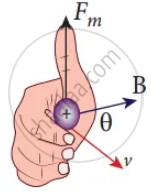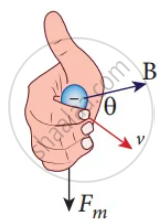Tamil Nadu Board of Secondary EducationHSC Science Class 12th

# Give an account of magnetic Lorentz force. - Physics

Numerical

Give an account of magnetic Lorentz force.

#### Solution

(a)(b)Direction of the Lorentz force on

(a) positive charge

(b) negative charge

1. An electric charge q is moving with velocity vec"v" in the magnetic field vec"B", experience a force called magnetic force vec"F"_"m"  deduced by Lorentz.
vec"F"_"m" = "q" (vec"v" xx vec"B") ....(1)
vec"F"_"m" = qvBsin θ ……………(2)

Equations (1) & (2) implies

2. vec"F"_"m" is directly proportional to the magnetic field vec"B".
3. vec"F"_"m" is directly proportional to the velocity vec"V".
4. vec"F"_"m" is directly proportional to sine of the angle between the velocity and magnetic field.
5. vec"F"_"m" is directly proportional to the magnitude of the charge q.
6. vec"F"_"m" is always perpendicular to vec"V" and vec"B".
7. The direction vec"F"_"m" on negative charge is opposite to the direction of vec"F"_"m" on positive charge.
8. If velocity vec"v" of the charge is along magnetic field, vec"F"_"m" = 0.
Concept: Lorentz Force
Is there an error in this question or solution?

#### APPEARS IN

Tamil Nadu Board Samacheer Kalvi Class 12th Physics Volume 1 and 2 Answers Guide
Chapter 3 Magnetism and magnetic effects of electric current
Evaluation | Q III. 14. | Page 192
Share